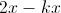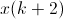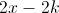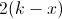# SSAT Middle Level Math : How to find the distributive property

## Example Questions

← Previous 1 3 4 5

### Example Question #1 : How To Find The Distributive Property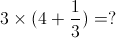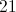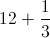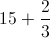Explanation:

Using the distributive property we get: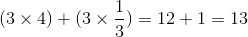### Example Question #1 : Distributive Property

Which of the following expressions is equivalent to the expression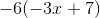?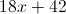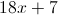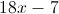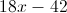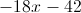Explanation:

By the distributive property of multiplication over addition,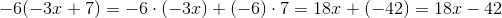### Example Question #1 : How To Find The Distributive Property

Which of the following expressions is equivalent to the expression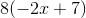?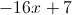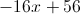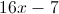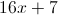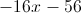Explanation:

By the distributive property of multiplication over addition,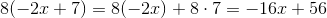### Example Question #1 : How To Find The Distributive Property

Simplify the expression: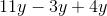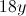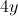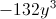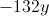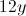Explanation:

Apply the distributive property: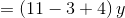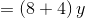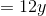### Example Question #2 : How To Find The Distributive Property

Simplify the expression: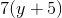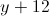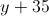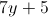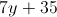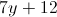Explanation:

Apply the distributive property: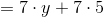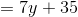### Example Question #1 : Distributive Property

Which of the following is an example of an application of the distributive property?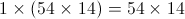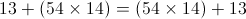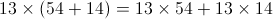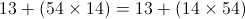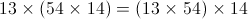Explanation:

According to the distributive property, for any values of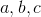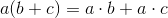If we set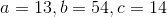, this become the statement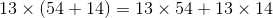,

so this is the correct choice.

All of the other statements are true for different reasons: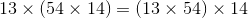is true because of the associative property of multiplication.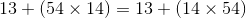is true because of the commutative property of multiplication.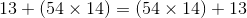is true because of the commutative property of addition.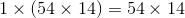is true because of the identity property of multiplication.

### Example Question #1 : How To Find The Distributive Property

Simplify the expression: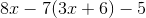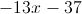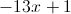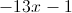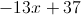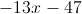Explanation:

Distribute, then collect like terms: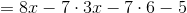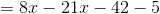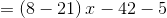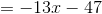### Example Question #1 : How To Find The Distributive Property

Diana is thirty-three years older than her son Colin, who is three times as old as her niece Sharon. Ifis Diana's age, how old is Sharon?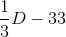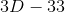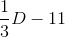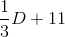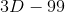Explanation:

Colin's age is thirty-three years less than Diana's age of, so Colin is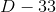years old; Sharon is one-third of this, or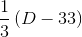. Using distribution, this can be rewritten as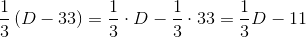.

### Example Question #1 : How To Find The Distributive Property

Nina is twenty-one years younger than her mother Caroline, who is one-third as old as their neighbor Mr. Hutchinson. Ifis Nina's age, how old is Mr. Hutchinson?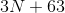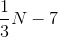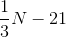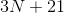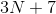Explanation:

Caroline is twenty-one years older than Nina, so her age is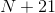. Mr. Hutchinson is three times as old as Caroline, so he is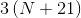. Using distribution, this can be rewritten as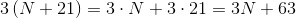.

### Example Question #1 : How To Find The Distributive Property

Which of the following expressions is equivalent to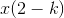?